# 3.3   Designing Data Types

In this section we discuss encapsulation, immutability, and inheritance, with particular attention to the use of these mechanisms in data-type design to enable modular programming, facilitate debugging, and write clear and correct code.

## Encapsulation.

The process of separating clients from implementations by hiding information is known as encapsulation. We use encapsulation to enable modular programming, facilitate debugging, and clarify program code.
• Complex numbers revisited.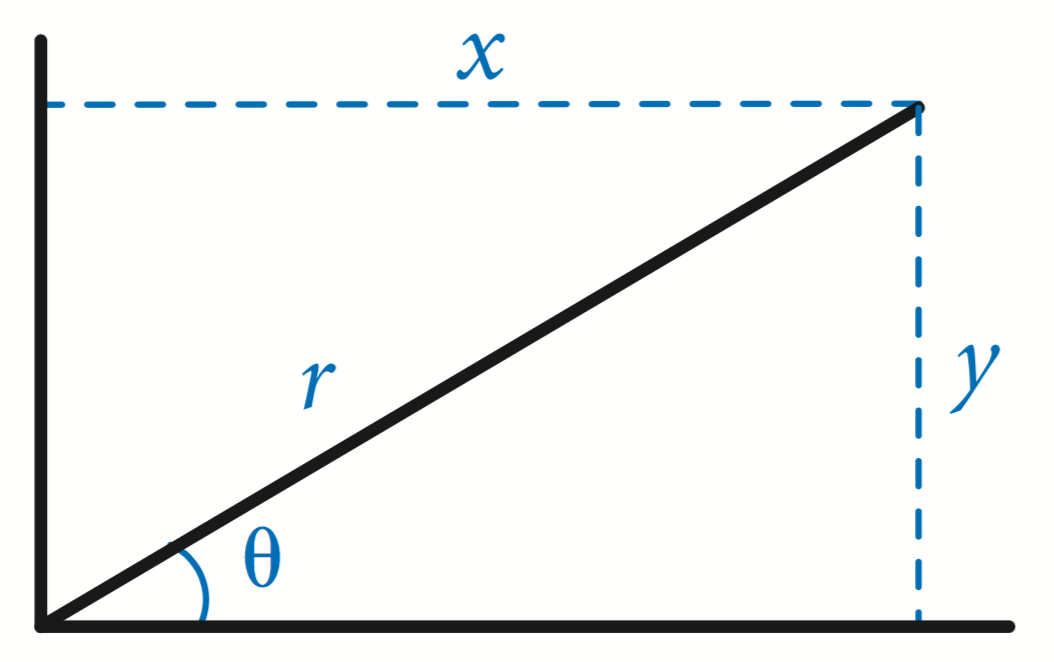Complex.java has the same API as Complex.java, except that it represents a complex number using polar coordinates $$r (\cos \theta + i \sin \theta)$$ instead of Cartesian coordinates as $$x + iy$$. The idea of encapsulation is that we can substitute one of these programs for the other without changing client code.
• Private. When you declare an instance variable (or method) to be private, you are making it impossible for any client (code in another class) to directly access that instance variable (or method). This helps enforce encapsulation.
• Limiting the potential for error. Encapsulation also helps programmers ensure that their code operates as intended. To understand the problem, consider Counter.java, which encapsulates a single integer and ensures that the only operation that can be performed on the integer is increment by 1.Without the private modifier, a client could write code like the following:
 Counter counter = new Counter("Volusia"); counter.count = -16022; 
With the private modifier, code like this will not compile.

## Immutability.An object from a data type is immutable if its data-type value cannot change once created. An immutable data type is one in which all objects of that type are immutable.
• Advantages of immutability. We can use immutable objects in assignment statements (or as arguments and return values from methods) without having to worry about their values changing. This makes immutable type easier to reason about and debug.
• Cost of immutability. The main drawback of immutability is that a new object must be created for every value.
• Final. When you declare an instance variable as final, you are promising to assign it a value only once. This helps enforce immutability.
• Reference types. The final access modifier does not guarantee immutability for instance variables of mutable types. In such cases, you must make a defensive copy.

## Spatial vectors.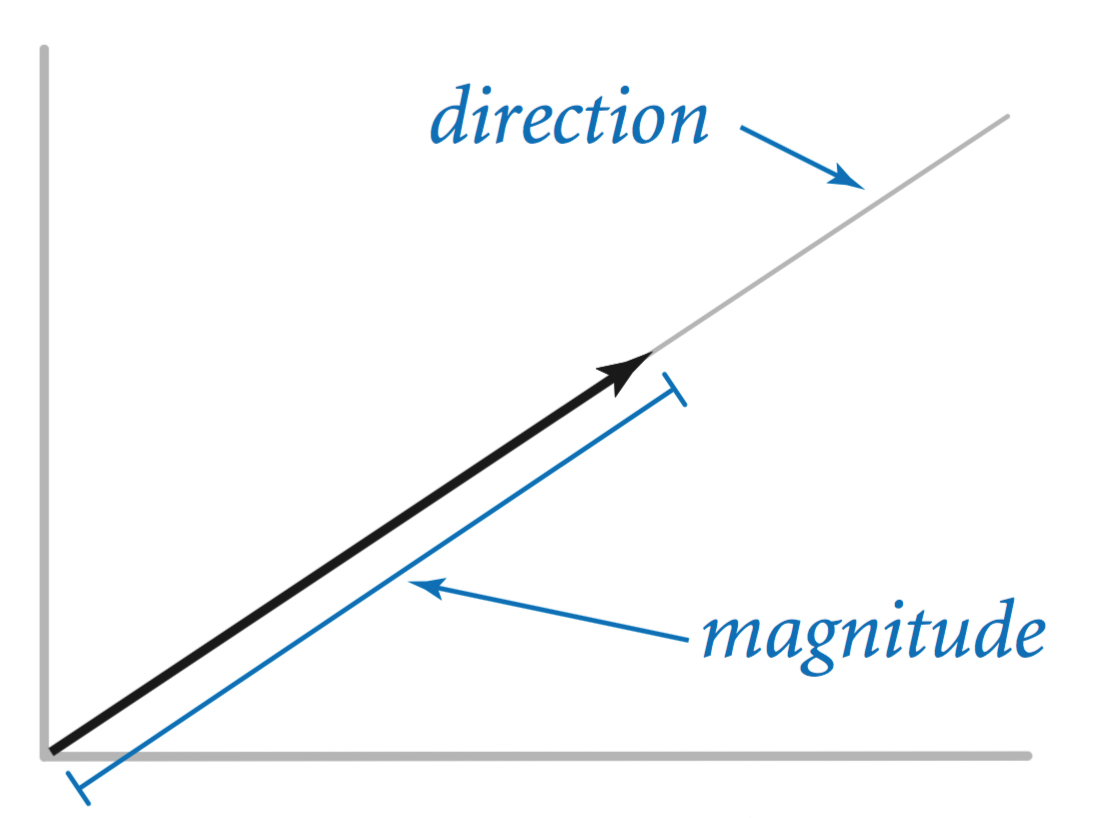A spatial vector is an abstract entity that has a magnitude and a direction. A sequence of n real numbers suffices to specify a vector in n-dimensional space. We use a boldface letter like $$\boldsymbol{x}$$ to denote the vector $$( x_0, x_1, \; \ldots, \; x_{n-1})$$.
• API. The basic operations on vectors are to add two vectors, multiply a vector by a scalar, compute the dot product of two vectors, and to compute the magnitude and direction, as follows:
• Addition: $$\boldsymbol{x} + \boldsymbol{y} = ( x_0 + y_0, x_1 + y_1, \; \ldots, \; x_{n-1} + y_{n-1})$$
• Vector scaling: $$\alpha \boldsymbol{x} = (\alpha x_0, \alpha x_1, \; \ldots, \; \alpha x_{n-1})$$
• Dot product $$\boldsymbol{x} \cdot \boldsymbol{y} = x_0y_0 + x_1y_1 + \, \ldots \, + x_{n-1}y_{n-1}$$
• Magnitude: $$\left | \boldsymbol{x} \right | = \sqrt{x_0^2 + x_1^2 + \, \ldots \, + x_{n-1}^2}$$
• Direction: $$\boldsymbol{x} \,/\, \left | \boldsymbol{x} \right | = (x_0 \,/\, \left | \boldsymbol{x} \right |, x_1 \,/\, \left | \boldsymbol{x} \right |, \; \ldots, \; x_{n-1} \,/\, \left | \boldsymbol{x} \right |)$$

These basic mathematical definitions lead immediately to an API: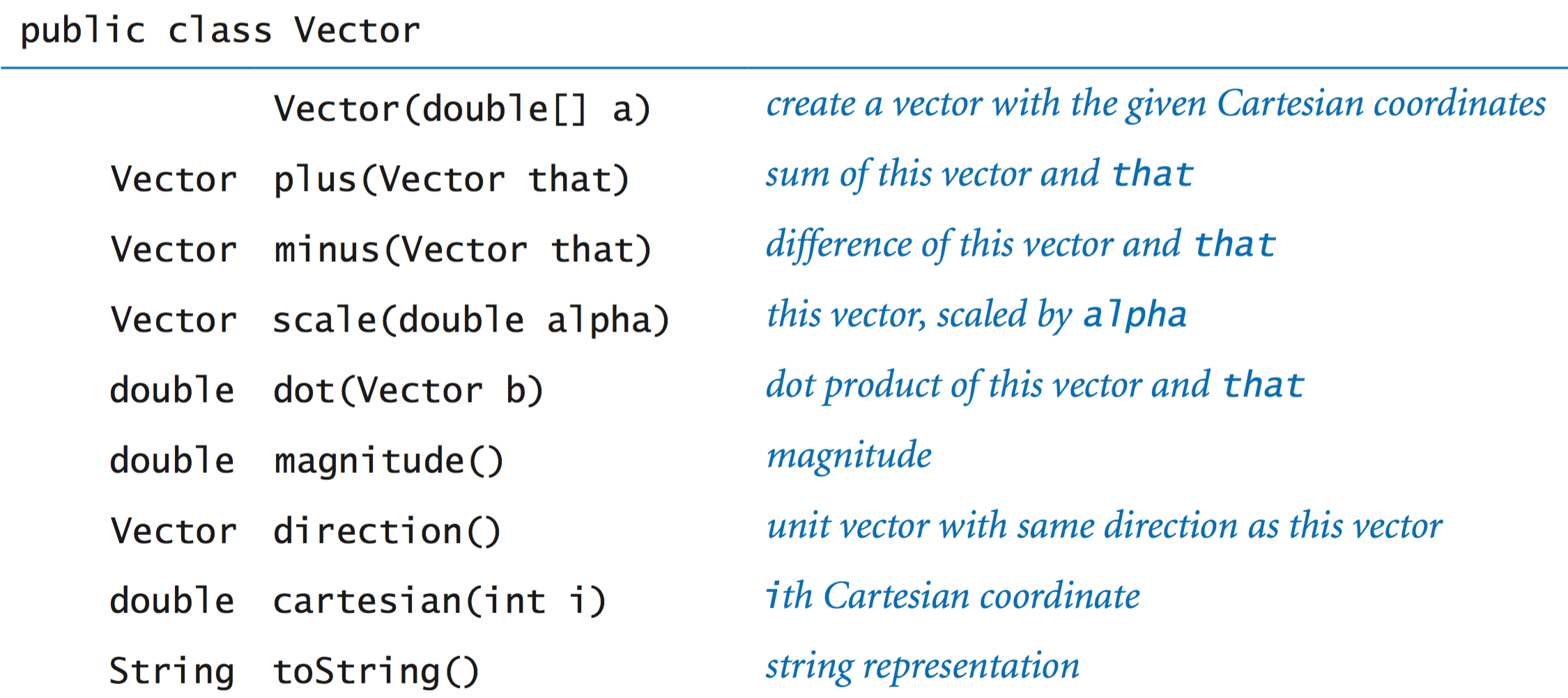• Implementation. Vector.java is an immutable data type that implements this API. Internally, it uses an array of length n to store the Cartesian coordinates.
• The this reference. Within an instance method (or constructor), the this keyword gives us a way to refer to the object whose instance method (or constructor) is being called. For example, the magnitude() method in Vector uses the this keyword in two ways: to invoke the dot() method and as the argument to the dot() method.
 // return the magnitude of this Vector public double magnitude() { return Math.sqrt(this.dot(this)); } 

## Interface inheritance (subtyping).

Java provides the interface construct for declaring a relationship between otherwise unrelated classes, by specifying a common set of methods that each implementing class must include. Interfaces enable us to write client programs that can manipulate objects of varying types, by invoking common methods from the interface.
• Defining an interface. Function.java defines an interface for real-valued functions of a single variable.
 public interface Function { public abstract double evaluate (double x); } 
The body of the interface contains a list of abstract methods. An abstract method is a method that is declared but does not include any implementation code; it contains only the method signature. You must save a Java interface in a file whose name matches the name of the interface, with a .java extension.
• Implementing an interface. To write a class that implements an interface, you must do two things.
• Include an implements clause in the class declaration with the name of the interface.
• Implement each of the abstract methods in the interface.

For example, Square.java and GaussianPDF.java implements the Function interface.

• Using an interface. An interface is a reference type. So, you can declare the type of a variable to be the name of an interface. When you do so, any object you assign to that variable must be an instance of a class that implements the interface. For example, a variable of type Function may store an object of type Square or GaussianPDF.
 Function f1 = new Square(); Function f2 = new GaussianPDF(); Function f3 = new Complex(1.0, 2.0); // compile-time error 
When a variable of an interface type invokes a method declared in the interface, Java knows which method to call because it knows the type of the invoking object. This powerful programming mechanism is known as polymorphism or dynamic dispatch.
• Plotting functions. FunctionGraph.java plots the graph of a real-valued function f in the interval [a, b] by sampling the function at n + 1 evenly spaced points. It works for any sufficiently smooth function f that implements the Function interface.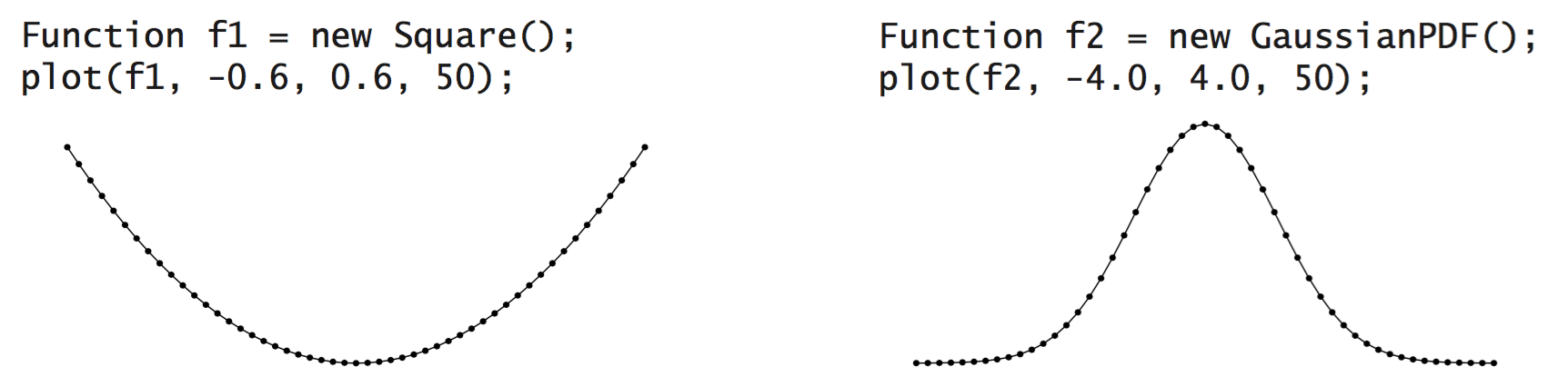• Numerical integration. RectangleRule.java estimates the integral of a positive real-valued function f in an interval (a, b) using the rectangle rule. It works for any sufficiently smooth function f that implements the Function interface.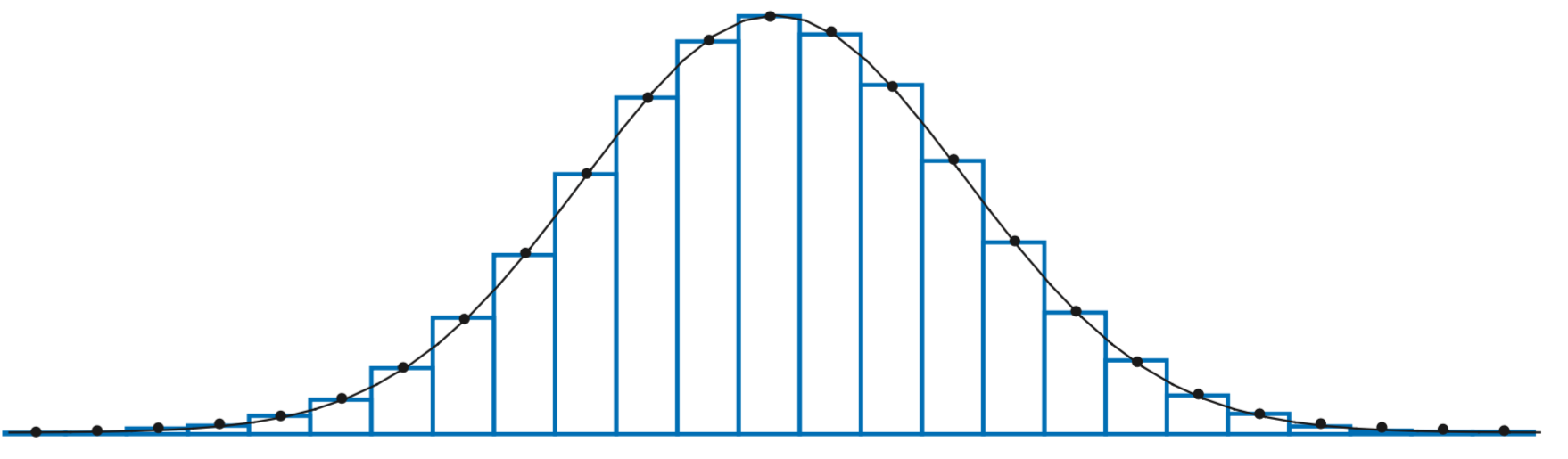• Lambda expressions. To simplify syntax, Java provides a powerful functional programming feature known as lambda expressions. You should think of a lambda expression as a block of code that you can pass around and execute later. In its simplest form, a lambda expression consists of the three elements:
• A list of parameters variables, separated by commas, and enclosed in parentheses
• The lambda operator ->
• A single expression, which is the value returned by the lambda expression

For example, the following lambda expression implements the hypotenuse function: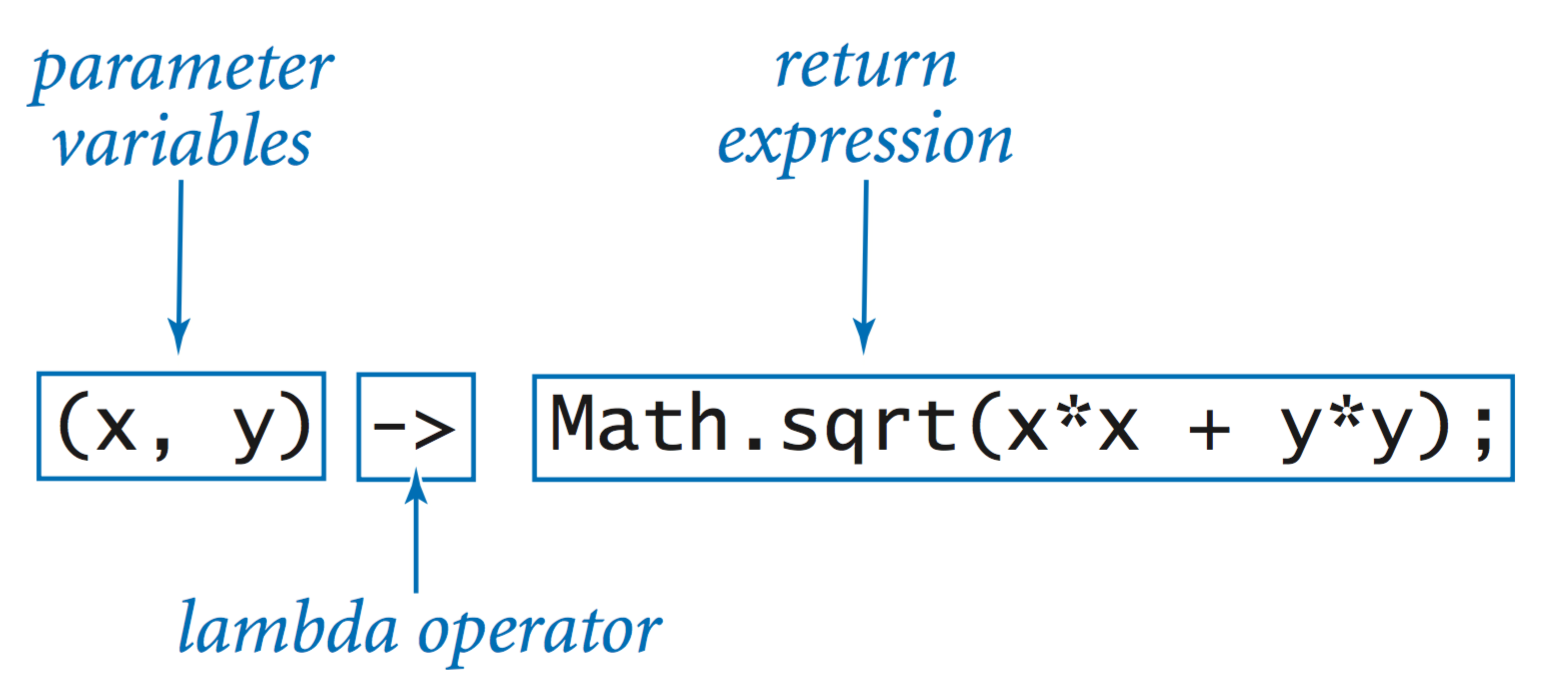Our primary use of lambda expressions is as a concise way to implement a functional interface (an interface with a single abstract method). Specifically, you can use a lambda expression wherever an object from a functional interface is expected. For example, all of the following expressions implement the Function.java interface: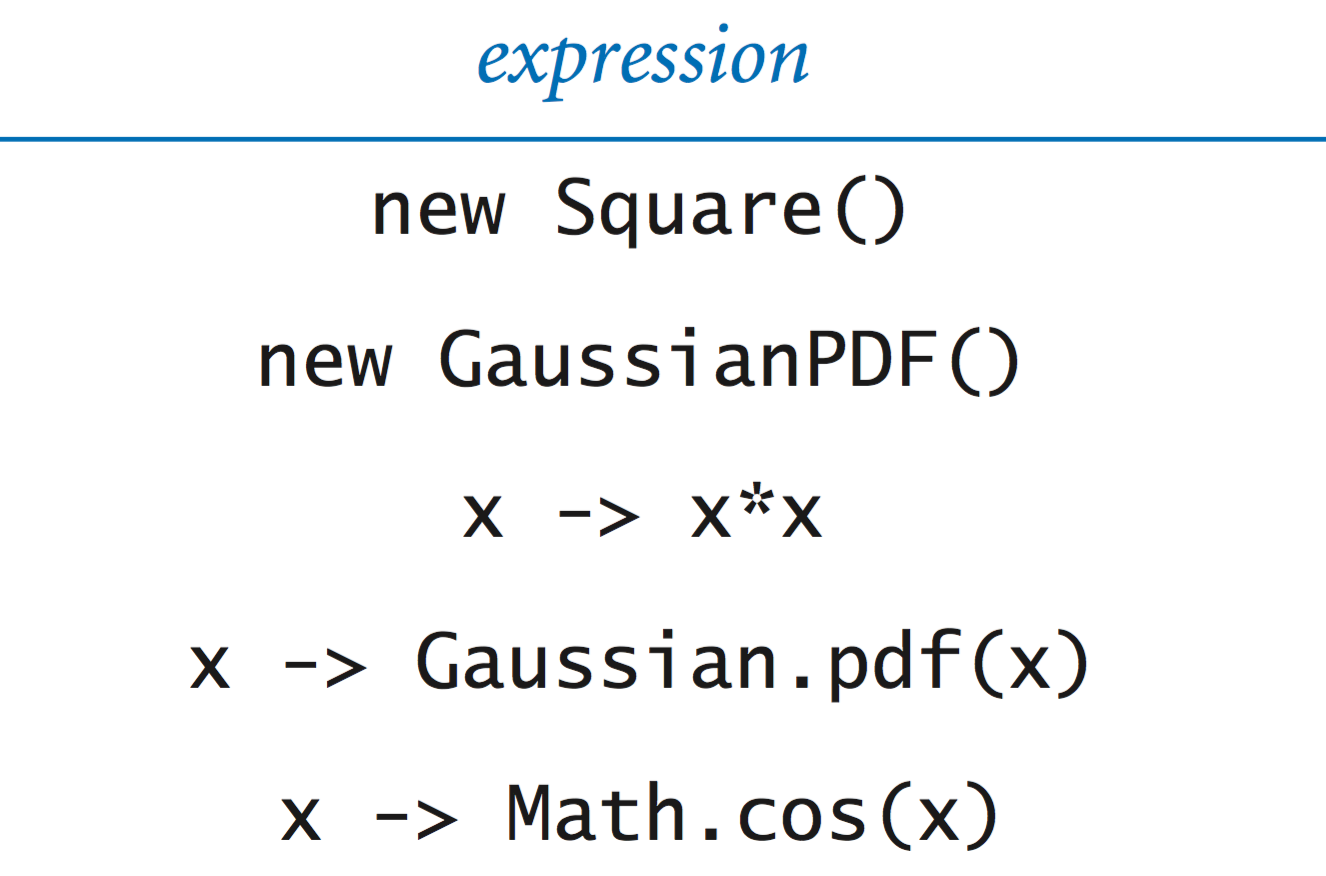Consequently, you can can integrate the square function with the call integrate(x -> x*x, 0, 10, 1000), bypassing the need to define a separate Square class.
• Built-in interfaces. Java includes three built-in interfaces that we will consider later this book.
• The interface java.util.Comparable defines an order in which to compare objects of the same type, such as alphabetical order for strings or ascending order for integers.
• The interfaces java.util.Iterator and java.lang.Iterable enable clients to iterate over the items in a collection, without relying on the underlying representation.

## Implementation inheritance (subclassing).

Java also supports another inheritance mechanism known as subclassing. The idea is to define a new class (subclass, or derived class) that inherits instance variables (state) and instance methods (behavior) from another class (superclass, or base class), enabling code reuse. Typically, the subclass redefines or overrides some of the methods in the superclass. For example, Java provides an elaborate inheritance hierarchy for GUI components: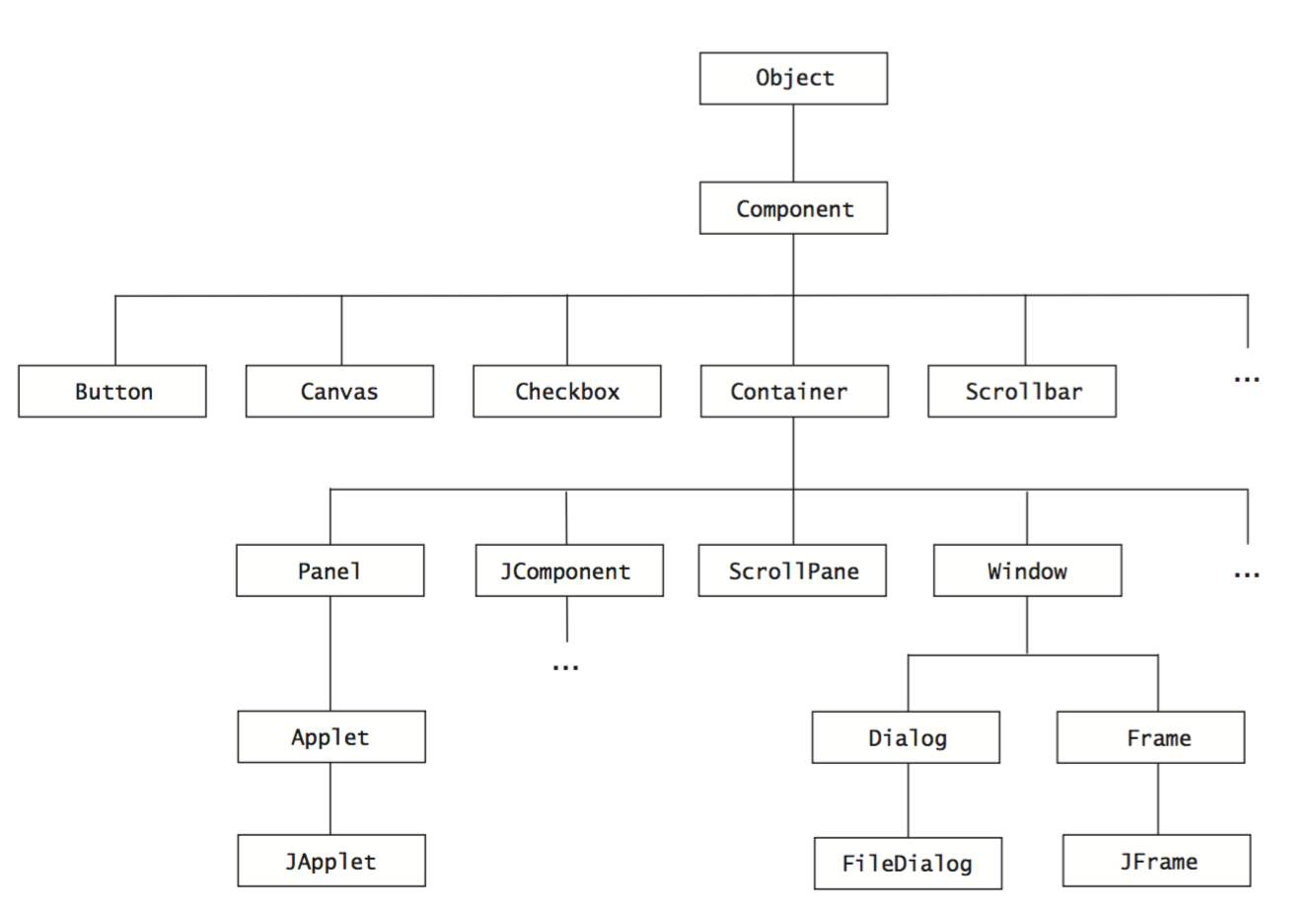In this book, we avoid subclassing because it works against encapsulation and immutability (e.g., the fragile base class problem and the circle–ellipse problem).
• Java's Object superclass. Certain vestiges of subclassing are built into Java and therefore unavoidable. Specifically, every class is a subclass of java.lang.Object. When programming in Java, you will often override one or more of these inherited methods: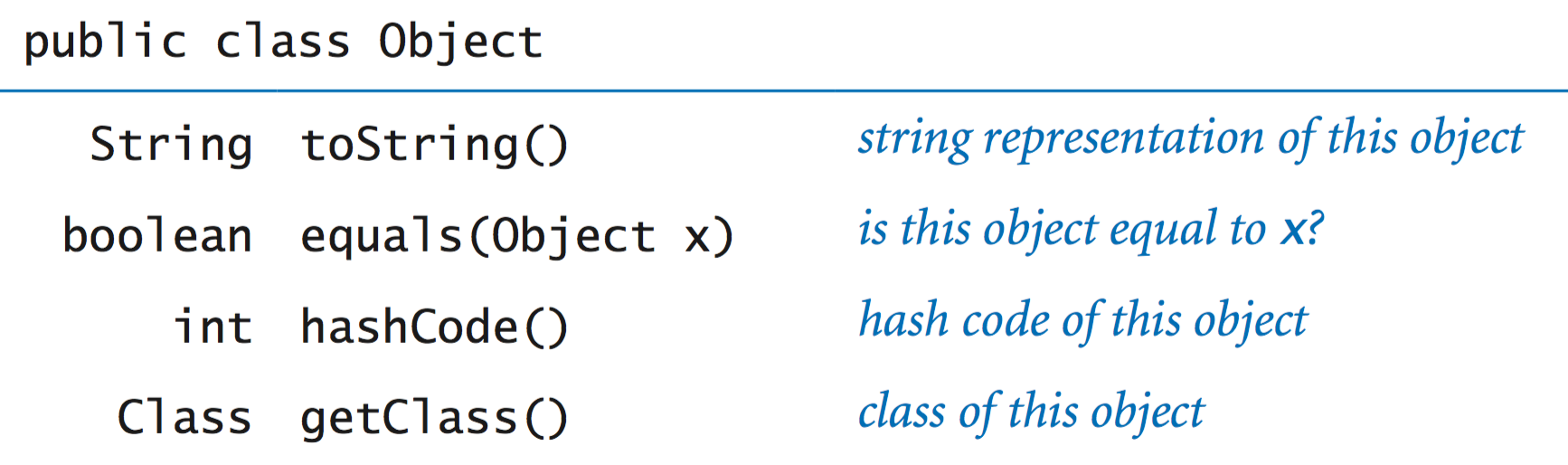• String conversion. Every Java class inherits the toString() method, so any client can invoke toString() for any object. This convention is the basis for Java’s automatic conversion of one operand of the string concatenation operator + to a string whenever the other operand is a string.
• Reference equality. If we test equality with (x == y), where x and y are object references, we are testing whether they have the same identity: whether the object references are equal.
• Object equality.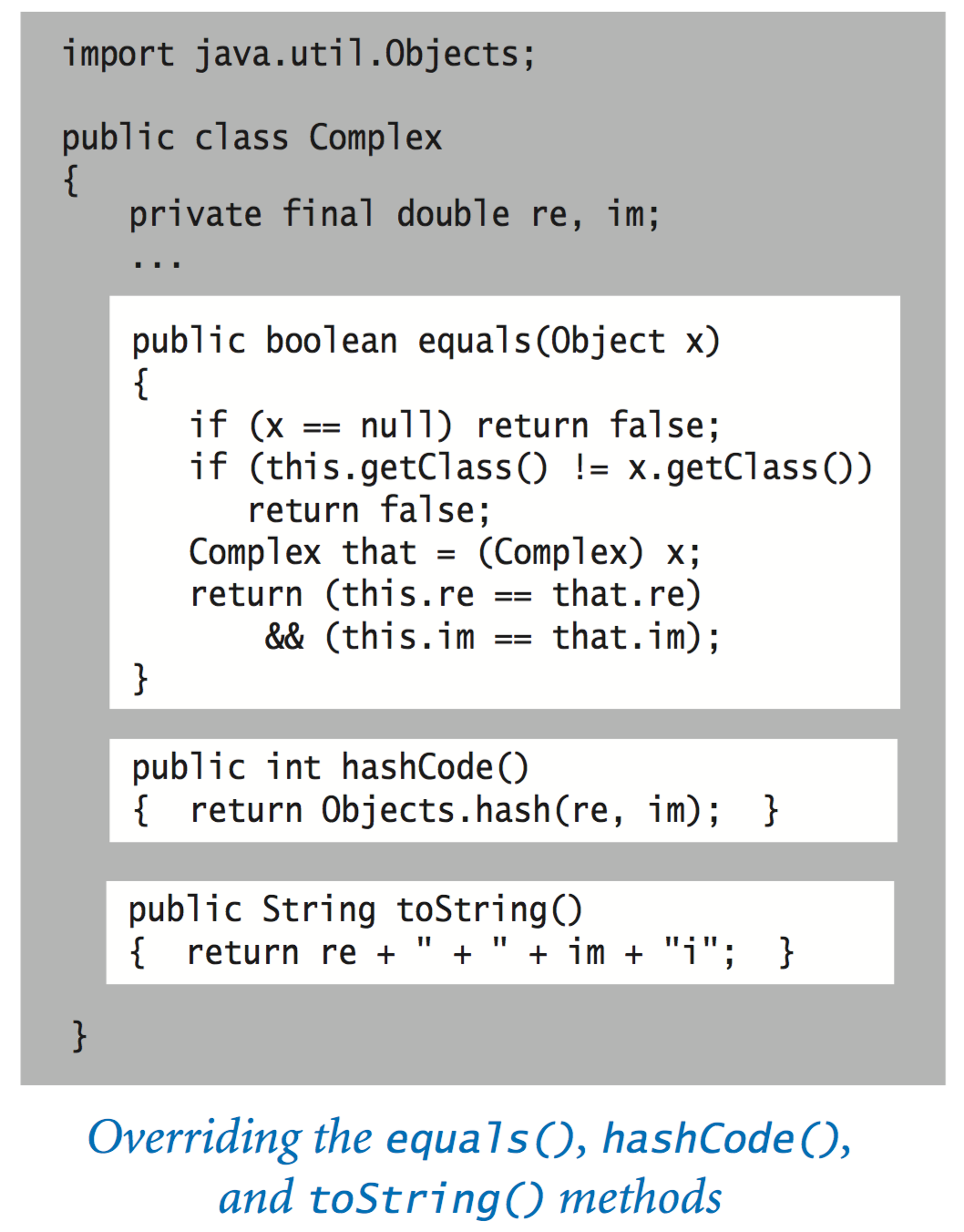The purpose of the equals() method is to test whether two objects are equal (correspond to the same data-type value). It must implement an equivalence relation:
• Reflexive: x.equals(x) is true.
• Symmetric: x.equals(y) is true if and only if y.equals(x) is true.
• Transitive: if x.equals(y) is true and y.equals(z) is true, then x.equals(z) is true.

In addition, the following two properties must hold:

• Multiple calls to x.equals(y) return the same truth value, provided neither object is modified between calls.
• x.equals(null) returns false.

Overriding the equals() method is unexpectedly intricate because its argument can be a reference to an object of any type (or null).

• Hashing. The purpose of the hashCode(), method is to support hashing, which is a fundamental operation that maps an object to an integer, known as a hash code. It must satisfy the following two properties:
• If x.equals(y) is true, then x.hashCode() is equal to y.hashCode().
• Multiple calls of x.hashCode() return the same integer, provided the object is not modified between calls.

Typically, we use the hash code to map an object x to an integer in a small range, say between 0 and m-1, using this hash function:

 private int hash(Object x) { return Math.abs(x.hashCode() % m); } 

Objects whose values are not equal can have the same hash function value but we expect the hash function to divide n typical objects from the class into m groups of roughly equal size.

• Wrapper types.The toString(), hashCode(), and equals() methods apply only to reference types, not primitive types. For example, the expression x.hashCode() works if x is a variable of type Integer but not if it is of type int. For situations where we wish want to represent a value from a primitive type as an object, Java supplies built-in reference types known as wrapper types, one for each of the eight primitive types.
• Autoboxing and unboxing. Java automatically converts between values from a wrapper type and the corresponding primitive type, so that you can write code like the following:
 Integer x = 17; // Autoboxing (int -> Integer) int a = x; // Unboxing (Integer -> int) 

## Application: data mining.

We consider a data mining application in which the goal is to associate with each document a vector known as a sketch so that so that documents that are different have sketches that are different and documents that are similar have sketches that are similar. Our API abstracts away this notion into the method similarTo(), which is a real number between 0 (not similar) and 1 (similar). The parameters k and d control the quality of the sketch.• Computing sketches.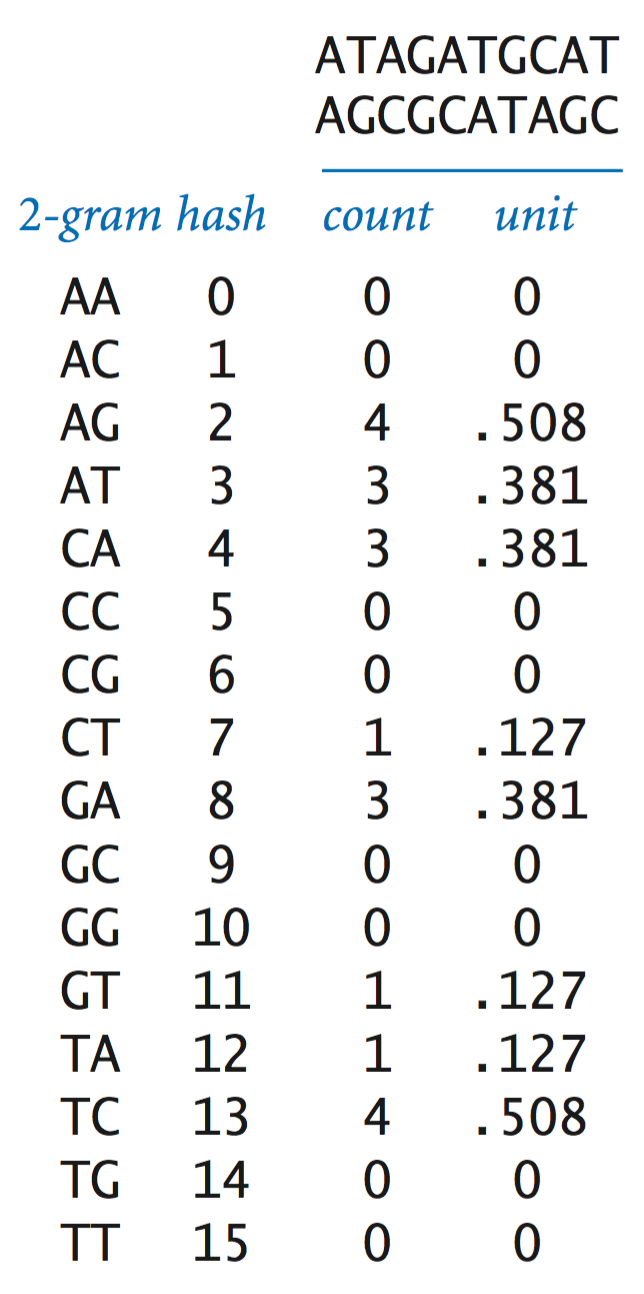Sketch.java uses a simple frequency count approach to compute the sketch of a text document. In its simplest form, it counts the number of time each k-gram (substring of length k) appears in the text. The sketch that we use is the direction of the vector defined by these frequencies.
• Hashing. For ASCII text strings there are 128 different possible values for each character, so there are 128k possible k-grams. For efficiency, Sketch.java uses hashing. That is, instead of counting the number of times each k-gram appears, we hash each k-gram to an integer between 0 and d−1 and count the number of times each hash value appears.
• Comparing sketches. Sketch.java uses the cosine similarity measure to compare two sketches:
$$x \cdot y = x_0y_0 + x_1y_1 + \; \ldots \; + x_{d-1}y_{d-1}$$
It is a real number between 0 and 1.
• Comparing all pairs. CompareDocuments.java prints the cosine similarity measure for all pairs of documents on an input list.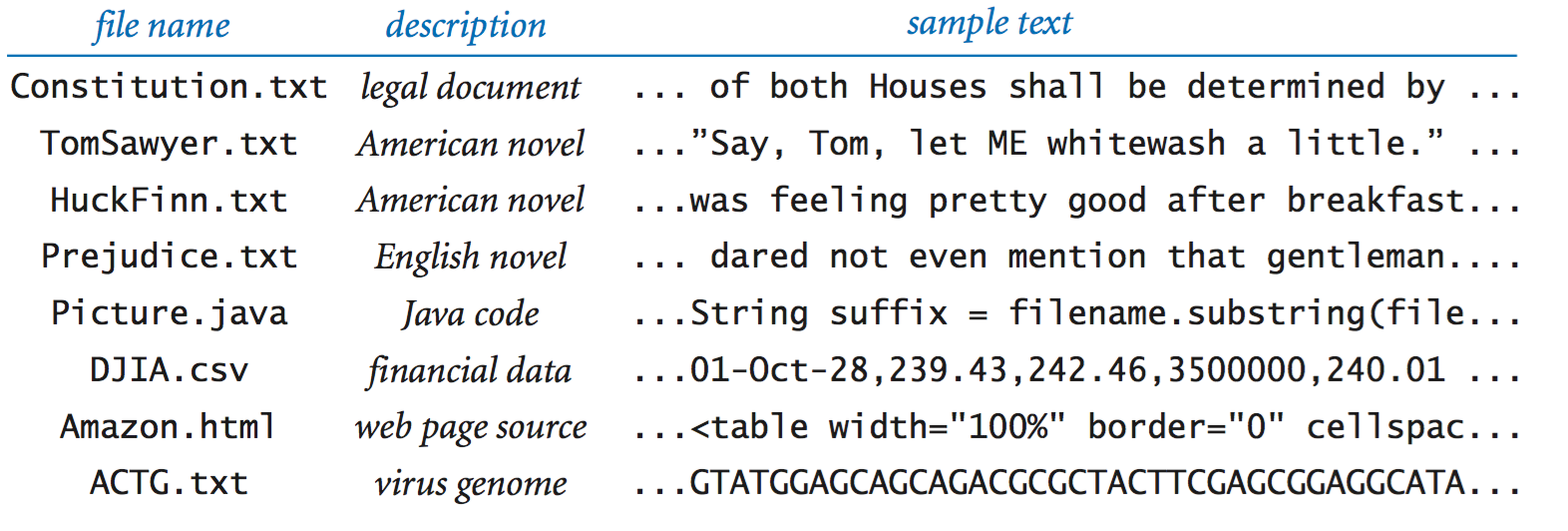## Design by contract.

We briefly discuss two Java language mechanisms that enable you to verify assumptions about your program while it is running—exceptions and assertions.
• Exceptions. An exception is a disruptive event that occurs while a program is running, often to signal an error. The action taken is known as throwing an exception. Java includes an elaborate inheritance hierarchy of predefined exceptions, several of which we have encountered previously.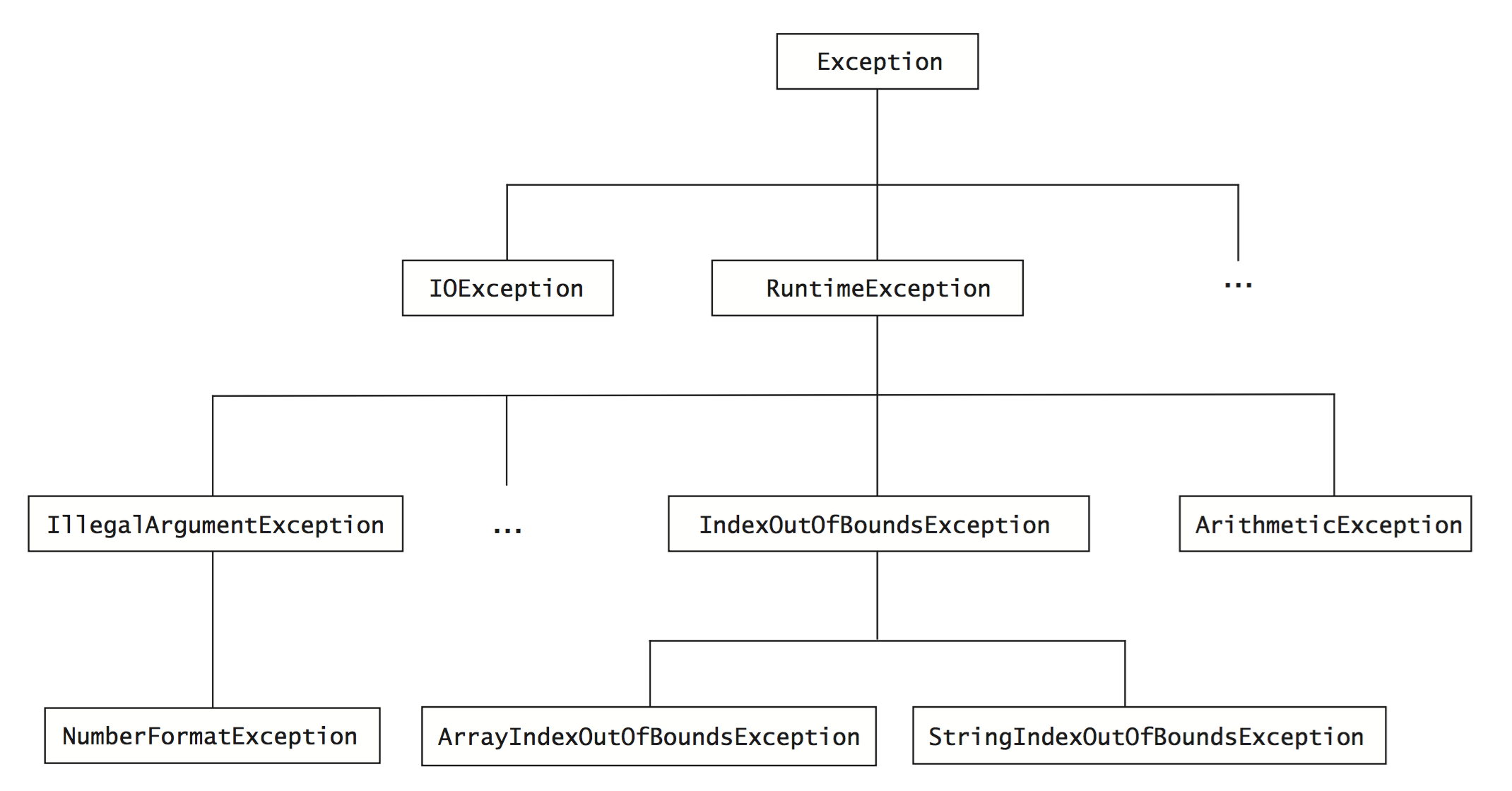It is good practice to use exceptions when they can be helpful to the user. For example, in Vector.java, we should throw an exception in plus() if the two vectors to be added have different dimensions:
 if (this.length() != that.length()) throw new IllegalArgumentException("Dimensions disagree."); 
• Assertions. An assertion is a boolean expression that you are affirming is true at some point during the execution of a program. If the expression is false, the program will throw an AssertionError, which typically terminates the program and reports an error message. For example, in Counter.java, we might check that the counter is never negative by adding the following assertion as the last statement in increment():
 assert count >= 0 : "Negative count detected in increment()"; 

By default, assertions are disabled, but you can enable them from the command line by using the -enableassertions flag (-ea for short). Assertions are for debugging only; your program should not rely on assertions for normal operation since they may be disabled.

In the design-by-contract model of programming, the designer expresses conditions about the behavior of the program using assertions.

• Precondition. A condition that the client promises to satisfy when calling a method.
• Postcondition. A condition that the implementation promises to achieve when returning from a method.
• Invariant. A condition that the implementation promises to satisfy while the method is executing.

#### Exercises

1. Give an implementation of minus() for Vector.java solely in terms of the other Vector methods, such as direction() and magnitude().

Solution:

 public Vector minus(Vector that) { return this.plus(that.scale(-1.0)); } 
2. Add a toString() method to Vector.java that returns the vector components, separated by commas, and enclosed in matching parentheses.

#### Creative Exercises

1. Statistics. Develop a data type for maintaining statistics for a set of real numbers. Provide a method to add data points and methods that return the number of points, the mean, the standard deviation, and the variance.

$$\begin{eqnarray*} \bar x &=& \frac{1}{n} \sum_i x_i \\ s^2 &=& \frac{\sum_i (x_i - \mu)^2}{n-1} \;\; = \;\; \frac{n \sum_i x_i^2 - (\sum_i x_i)^2}{n(n-1)} \end{eqnarray*}$$

Develop two implementations: OnePass.java whose instance values are the number of points and the sum of the values, and the sum of the squares of the values, TwoPass.java that keeps an array containing all the points. For simplicity, you may take the maximum number of points in the constructor. Your first implementation is likely to be faster and use substantially less space, but is also likely to be susceptible to roundoff error.

Solution: StableOnePass.java is a well-engineered alternative that is is numerically stable and does not require an array to store the elements.

$$\begin{eqnarray*} m_0 &=& 0 \\ s_0 &=& 0 \\ m_n &=& m_{n-1} + \frac{1}{n} \; (x_n - m_{n-1}) \\ s_n &=& s_{n-1} + \frac{n-1}{n} \; (x_n - m_{n-1})^2 \\ \bar x &=& m_n \\ s^2 &=& \frac{1}{n-1} s_n \end{eqnarray*}$$
2. Genome. Develop a data type to store the genome of an organism. Biologists often abstract away the genome to a sequence of nucleotides (A, C, G, or T). The data type should support the method addNucleotide(), nucleotideAt(), as well as isPotentialGene(). Develop three implementations.
• Use one instance variable of type String, implementing addCodon() with string concatenation. Each method call takes time proportional to the length of the current genome.
• Use an array of characters, doubling the length of the array each time it fills up.
• Use a boolean array, using two bits to encode each codon, and doubling the length of the array each time it fills up.

Solution: StringGenome.java, Genome.java, and CompactGenome.java.

3. Encapsulation. Is the following class immutable?
 import java.util.Date public class Appointment { private Date date; private String contact; public Appointment(Date date) { this.date = date; this.contact = contact; } public Date getDate() { return date; } 
Solution: No, because Java's java.util.Date is mutable. To correct, make a defensive copy of the date in the constructor and make a defensive copy of the date before returning to the client.
4. Date. Design an implementation of Java's java.util.Date API that is immutable and therefore corrects the defects of the previous exercise.

Partial solution: Date.java.

#### Web Exercises

1. Add methods to Genome.java to test for equality and return the reverse-complemented genome.
2. Add methods to Date.java to check which season (Spring, Summer, Fall, Winter) or astrological sign (Pisces, Libra, ...) a given date lies. Be careful about events that span December to January.
3. Add a method daysUntil() to Date.java that takes a Date as an argument and returns the number of days between the two dates.
4. Create an implementation Date2.java that represents a date a single integer that counts the number of days since January 1, 1970. Compare to Date.java.
5. Create a Rectangle ADT that represents a rectangle. Represent a rectangle by two points. Include a constructor, a toString method, a method for computing the area, and a method for drawing using our graphics library.
6. Repeat the previous exercise, but this time represent a Rectangle as the lower left endpoint and the width and height.
7. Repeat the previous exercise, but this time represent a Rectangle as the center and the width and height.
8. Sparse vector. Create a data type for sparse vectors. Represent a sparse vector by an array of indices (of nonzeros) and a parallel array of the corresponding nonzero values. Assume the indices are in ascending order. Implement the dot product operation.
9. Copy constructor. Only needed if data type is mutable. Otherwise, assignment statement works as desired.
 public Counter(Counter x) { count = x.count; } Counter counter1 = new Counter(); counter1.hit(); counter1.hit(); counter1.hit(); Counter counter2 = new Counter(counter1); counter2.hit(); counter2.hit(); StdOut.println(counter1.get() + " " + counter2.get()); // 3 5 
10. Define an interface DifferentiableFunction.java for twice-differentiable function. Write a class Sqrt.java that implements the function f(x) = c - x^2.
11. Write a program Newton.java that implements Newton's method to find a real root of a sufficiently smooth function, given that you start sufficiently close to a root. When method converges, it does so quadratically. Assume that it takes a DifferentiableFunction as argument.
12. Generating random numbers. Different methods to generate a random number from the standard Gaussian distribution. Here, encapsulation enables us to replace one version with another that is more accurate or efficient. Trigonometric method is simple, but may be slow due to calling several transcendental functions. More importantly, it suffers from numerical stability problems when x1 is close to 0. Better method is alternate form of Box-Muller method. reference. Both methods require two values from a uniform distribution and produce two values from the Gaussian distribution with mean 0 and standard deviation 1. Can save work by remembering the second value for the next call. (This is how it is implemented in java.util.Random.) Their implementation is the polar method of Box-Muller, saving the second random number for a subsequent call. (See Knuth, ACP, Section 3.4.1 Algorithm C.)
13. LAX airport shutdown. On September 14, 2004 Los Angeles airport was shut down due to software breakdown of a radio system used by air traffic controllers to communicate with pilots. The program used a Windows API function call GetTickCount() which returns the number of milliseconds since the system was last rebooted. The value is returned as a 32 bit integer, so after approximately 49.7 days it "wraps around." The software developers were aware of the bug, and instituted a policy that a technician would reboot the machine every month so that it would never exceed 31 days of uptime. Oops. LA Times blamed the technician, but the developers are more to blame for shoddy design.
14. Polar representation of points. Re-implement the Point.java data type using polar coordinates.

Solution: PointPolar.java.

15. Spherical coordinates. Represent a point in 3D space using Cartesian coordinates $$(x, y, z)$$ or spherical coordinates $$(r, \theta, \phi)$$. To convert from one to the other, use

$$\begin{array}{lllllll} r &=& \sqrt{x^2 + y^2 + z^2} &\hspace{.3in} & x &=& r \cos \theta \sin \phi \\ \theta &=& \tan^{-1}(y/x) & & y &=& r \sin \theta \sin \phi \\ \phi &=& \cos^{-1}(z/r) & & z &=& r \cos \phi \\ \end{array}$$
16. Colors. Can represent in RGB, CMYK, or HSV formats. Natural to have different implementations of same interface.
17. ZIP codes. Implement an ADT that represents a USPS ZIP code. Support both the original 5 digit format and the newer (but optional) ZIP+4 format.